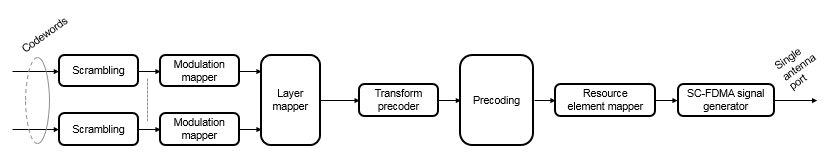ltePSSCHPRBS

PSSCH pseudorandom binary scrambling sequence

Description

example

[seq,cinit] = ltePSSCHPRBS(ue,n) returns the first n outputs of the PSSCH pseudorandom binary scrambling sequence (PRBS) for the specified UE settings structure. It also returns an initialization value cinit for the pseudorandom binary sequence (PRBS) generator.

The scrambling sequence generated should be applied to the coded PSSCH data carried by the associated subframe. The PRBS sequence generator used is initialized with ${c}_{\text{init}}={n}_{\text{ID}}^{\text{X}}×{2}^{14}+{n}_{\text{ssf}}^{\text{PSSCH}}×{2}^{9}+510$. For more information, see Physical Sidelink Shared Channel Processing, ue.NSAID and ue.NXID

example

[seq,cinit] = ltePSSCHPRBS(ue,n,mapping) specifies the format of the returned sequence, seq, through the mapping input.

[subseq,cinit] = ltePSSCHPRBS(ue,pn) returns a subsequence of a full PRBS sequence, specified by pn.

[subseq,cinit] = ltePSSCHPRBS(ue,pn,mapping) specifies the format of the returned subsequence, subseq, through the mapping input.

Examples

collapse all

Scramble a PSSCH codeword by generating the PSSCH pseudorandom binary sequence (PRBS) and applying an exclusive OR operation on the two sequences.

Create a UE settings structure with required fields. Generate the required length of the PRBS. Scramble the PSSCH codeword with the PRBS sequence using xor.

ue = struct('NSAID',255,'NSubframePSSCH',0);

codeword = ones(1152,1);
psschPrbs = ltePSSCHPRBS(ue,length(codeword));

scrambled = xor(psschPrbs,codeword);

Scramble PSSCH Codeword

• Generate the required length of the PRBS.

• Scramble the PSSCH codeword with the PRBS sequence using xor.

• Modulate the logical scrambled data.

ue = struct('NSAID',255,'NSubframePSSCH',0);

codeword = ones(1152,1);
psschPrbs = ltePSSCHPRBS(ue,length(codeword));

scrambled = xor(psschPrbs,codeword);

txsym = lteSymbolModulate(scrambled,'16QAM');

Descramble Recovered Codeword

• Generate the PSSCH PRBS in signed form.

• Descramble the vector representing a sequence of soft bits by generating the PSSCH PRBS in signed form and performing a pointwise multiplication between the PRBS sequence and the recovered soft data.

• Compare the transmitted codeword to the recovered codeword.

sym = awgn(txsym,30,'measured');
softdata = lteSymbolDemodulate(sym,'16QAM');

scramblingSeq = ltePSSCHPRBS(ue,length(softdata),'signed');
descrambled = softdata.*scramblingSeq;

isequal(codeword,descrambled > 0)
ans = logical
1

The transmitted codeword matches the hard decision on the descrambled data.

Input Arguments

collapse all

User equipment settings, specified as a parameter structure containing these fields:

Sidelink mode, specified as 'D2D' or 'V2X'.

Data Types: char | string

Sidelink group destination identity, specified as an integer in the interval [0, 255].

This field is the lower eight bits of the full 24-bit ProSe Layer-2 group destination ID. This field and the NSubframePSSCH field control the value of the scrambling sequence at the start of each subframe. This field is required only for D2D sidelink.

Data Types: double

V2X scrambling identity, specified as an integer scalar. NXID is the 16 bit CRC associated with the PSCCH SCI grant. It is only required for V2X sidelink.

Data Types: double

PSSCH subframe number in the PSSCH subframe pool, specified as an integer scalar. (${n}_{\text{ssf}}^{\text{PSSCH}}$)

NSubframePSSCH and NSAID control the values of the scrambling sequence. It is only required for D2D sidelink.

Data Types: double

Data Types: struct

Number of elements in returned sequence, seq, specified as a numeric scalar.

Data Types: double

Range of elements in returned subsequence, subseq, specified as a row vector of [p n]. The subsequence returns n values of the PRBS generator, starting at position p (0-based).

Data Types: double

Output sequence formatting, specified as 'binary' or 'signed'.

• 'binary' maps true to 1 and false to 0.

• 'signed' maps true to –1 and false to 1.

Data Types: char | string

Output Arguments

collapse all

PSSCH pseudorandom scrambling sequence, returned as a logical column vector or a numeric column vector. seq contains the first n outputs of the physical sidelink shared channel (PSSCH) scrambling sequence. If you set mapping to 'signed', the output data type is double. Otherwise, the output data type is logical.

Data Types: logical | double

PSSCH pseudorandom scrambling subsequence, returned as a logical column vector or a numeric column vector. subseq contains the values of the PRBS generator specified by pn. If you set mapping to 'signed', the output data type is double. Otherwise, the output data type is logical.

Data Types: logical | double

Initialization value for PRBS generator, returned as a numeric scalar.

Data Types: uint32

collapse all

Physical sidelink shared channel (PSSCH) processing includes PSSCH-specific scrambling, QPSK or 16-QAM modulation, and SC-FDMA transform precoding. PSSCH processing follows the processing steps used for PUSCH, with variations defined in TS 36.211, Section 9.3.For PSSCH, the input codeword length is Mbits = NRE × Nbps, where Nbps is the number of bits per symbol. PSSCH modulation is either QPSK (2 bits per symbol) or 16 QAM (4 bits per symbol).

The number of PSSCH resource elements (NRE) in a subframe is NRE = NPRB × NREperPRB × NSYM and includes symbols associated with the sidelink SC-FDMA guard symbol.

• NPRB is the number of physical resource blocks (PRB) used for transmission.

• NREperPRB is the number of resource elements in a PRB. Each PRB has 12 resource elements.

• NSYM is the number of SC-FDMA symbols in a PSSCH subframe, including symbols associated with the sidelink SC-FDMA guard symbol. The number of SC-FDMA symbols in a PSSCH subframe is 12 for D2D normal cyclic prefix or 10 for D2D extended cyclic prefix and V2X.

The info structure output by ltePSSCHIndices provides Mbits and NRE as info.G and info.Gd respectively.

The scrambling sequence generator is initialized with ${c}_{\text{init}}={n}_{\text{ID}}^{\text{X}}×{2}^{14}+{n}_{\text{ssf}}^{\text{PSSCH}}×{2}^{9}+510$ at the start of every PSSCH subframe. For D2D sidelink, ${n}_{\text{ID}}^{\text{SA}}$ is the destination identity (NSAID) obtained from the sidelink shared channel. For V2X, ${n}_{\text{ID}}^{\text{SA}}$ is the V2X scrambling identity (NXID). ${n}_{\text{ssf}}^{\text{PSSCH}}$ is the subframe number in the PSSCH subframe pool (NSubframePSSCH).

ltePSSCH requires CyclicPrefixSL to deduce the number of resource blocks allocated for SC-FDMA precoding symbols.

 3GPP TS 36.211. “Evolved Universal Terrestrial Radio Access (E-UTRA); Physical Channels and Modulation.” 3rd Generation Partnership Project; Technical Specification Group Radio Access Network. URL: https://www.3gpp.org.